(Last Updated On: July 19, 2022)

In the exploration of eLearning era, there is an increasing number of fields that have their online courses designed. When it comes to Natural Science subjects, many designers find it challenging to write equations effectively. A research published in 2005 showed that students attrition rates are higher in eLearning. It also indicated: “E-learning systems are poorly adapted to mathematics”. Hence, creating a mathematics, physics or chemistry course becomes a daunting task.  However, it is what happened in the past. The world changes non-stop and eLearning is not out of that alteration. So, what can we do to overcome this dilemma? What can help us work excellently with the mathematical equation in designing online courses?

Now, let’s discuss in detail to find the matter’s solution.Photo by Public Domain Pictures from Pexels

## Why Equation is a Necessary Feature of all eLearning software?

The famous mathematician Edward Frenkel once said: “Mathematics directs the flow of the universe, lurks behind its shapes and curves, holds the reins of everything from tiny atoms to the biggest stars”. As you might know, Mathematics seems to get more complicated when you begin doing equations and using symbols like α or ϵ instead of numbers. However, equations are really important for calculating the same result many times with different variables. Here are some common mathematical formulas in Math, Physics, Chemistry, and Biology: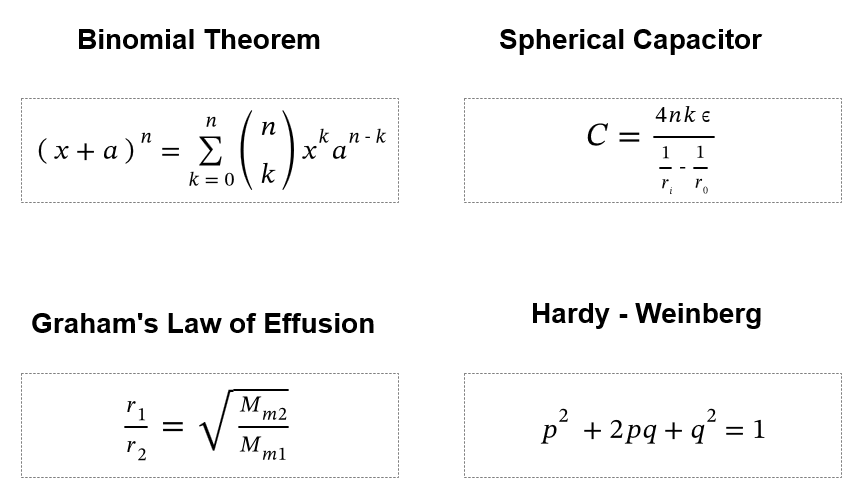These formulas may make you confused to create by a computer. Thus, if an eLearning authoring tool allows inserting those formulas, online courses will be created with ease. In other words, the Equation is a vital feature that cannot be lacking in any eLearning authoring tools.

## How to Write an Equation Effectively in any eLearning Course?

There are various eLearning tools in the market at present. In fact, not all of them supports equation function. Some tools suggest that users can take equation photo then insert it to the course design. However, this takes time, even becoming a tough task. Imagine that the formula photo appears above or below the baseline. So, what will you do to make equation in line with the text? This problem comes to a deadlock when the answer of the mathematical formula is an equation as well.  In other cases, people tend to use the characters available on the keyboard to express their ideas. For example, they would use “cos ^ 2” to represent cos squared. Nevertheless, actual situations are more complicated than the simple previous example. The reason is that there are several equations containing letters or symbols that are unavailable on your keyboard.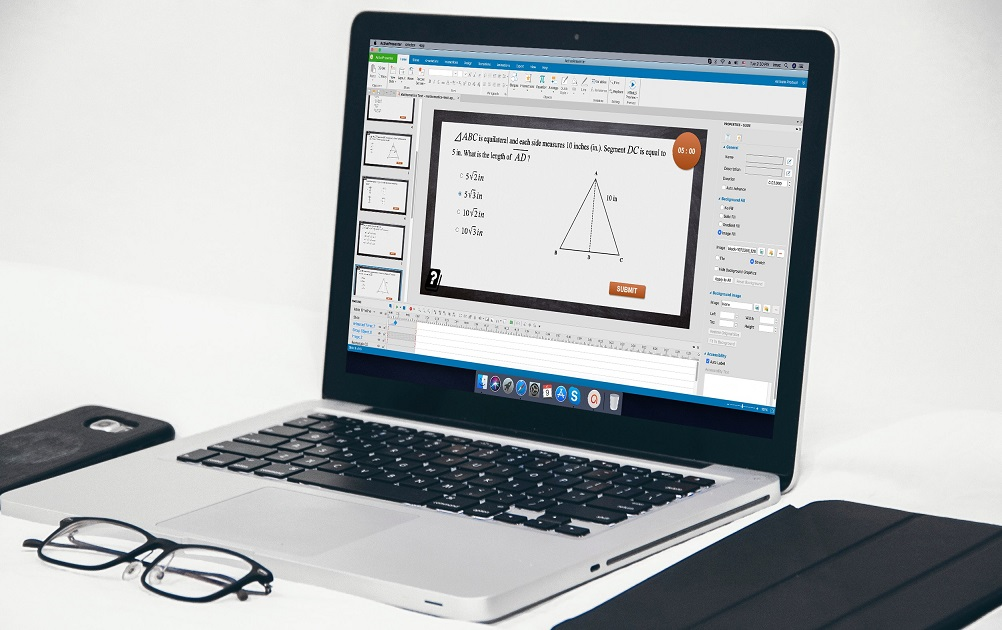Understanding the difficulty of online courses’ designers, ActivePresenter brought out Equation feature. Indeed, it makes ActivePresenter more outstanding than so many eLearning authoring tools in the market. This highlight feature helps you work perfectly with mathematical formulas, even the most difficult ones. From now on, you can create so many Natural Science courses easily. Thus, let’s get an inside look at this helpful feature.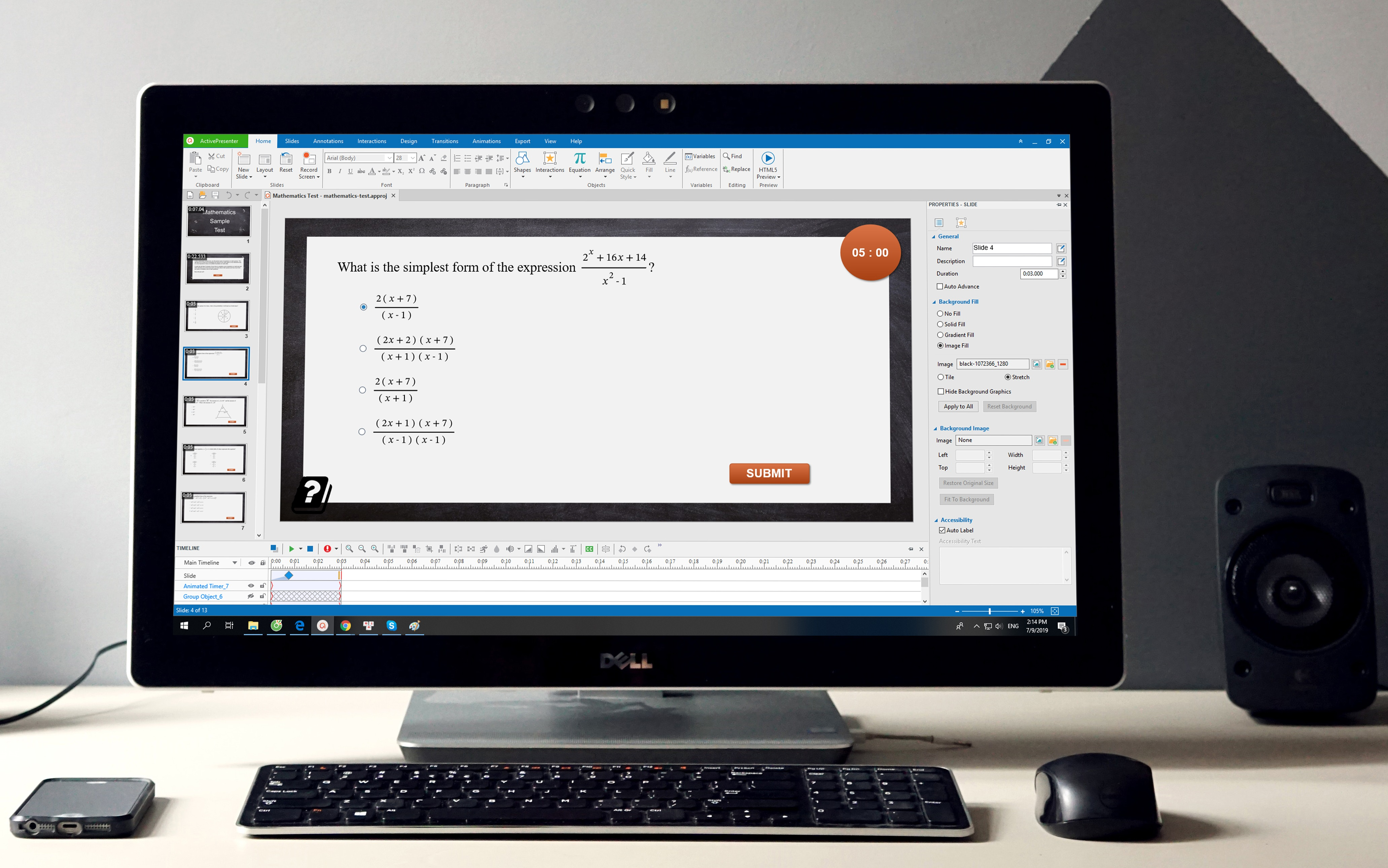### Equation Feature in ActivePresenter

In ActivePresenter, the Equation feature looks like pi (π) symbol. It can be found in both the Home tab and Insert tab. So, you can recognize it effortlessly for the first time you open ActivePresenter app.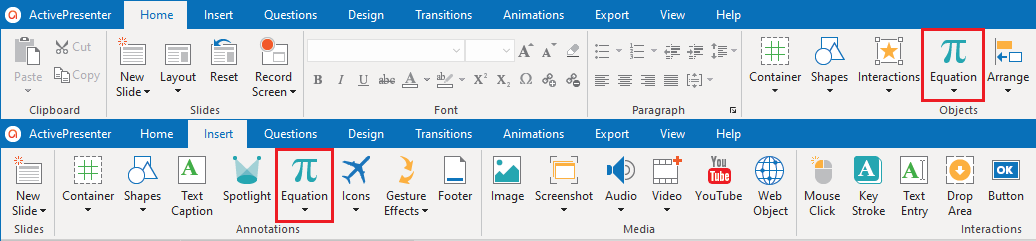What’s more, ActivePresenter supplies both list of built-in equations and the chance for yourself to write equations effectively. There are 9 most common types of the equation: Area of Circle, Binomial Theorem, Expansion of a Sum, Fourier Series, Pythagorean Theorem, Quadratic Formula, Taylor Expansion, Trig Identity 1, and Trig Identity 2. Surely, you will feel inserting formulas is just a breeze with ActivePresenter.
When creating an online course, you may need more than the available mathematical formulas. ActivePresenter gives you an alternative solution. It allows you to not only insert equations but also build up your own one by using a library of math symbols. Insert New Equation function is placed at the bottom of the equations list. This function contains a range of symbols and structures. Please refer to

Now, let’s take an example. This is one of 17 Math Equations that Changed the World created with ActivePresenter.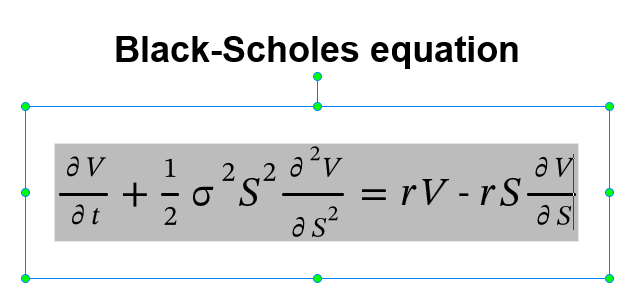As you can see, this equation is displayed by text. That’s the reason why it looks clear and sharp with high quality, even when you zoom or resize it. Meanwhile, you cannot perform like that with an equation photo.

## Final WordIn the previous blog post, you have seen why ActivePresenter is the best choice for eLearning design. Today’s post has given you one more reason to companion with ActivePresenter. Are you persuaded? Download ActivePresenter and try this feature to speed up your workflow. Have any problems? Feel free to contact us. We are always ready to support you!

More articles:

Writing a Script for Video Effectively: Five Secrets Every Beginners Should Know